Bihar Board Class 10th Maths Solutions Chapter 2 Polynomials Ex 2.2

Bihar Board Class 10th Maths Solutions Chapter 2 Polynomials Ex 2.2 Textbook Questions and Answers.

BSEB Bihar Board Class 10th Maths Solutions Chapter 2 Polynomials Ex 2.2

Question 1.
Find the zeroes of the following quadratic polynomials and verify the relationship between the zeroes and the coefficients:

1. x2 – 2x – 8
2. 4s2 – 4s + 1
3. 6x2 – 3 – 7x
4. 4u2 + 8u
5. t2 – 15
6. 3x2 – x – 4

Solution:
1. We have:
x2 – 2x – 8 = x2 + 2 – 4x – 8
= x(x + 2) – 4(x + 2)
= (x + 2)(x – 4)
The value of x2 – 2x – 8 is 0, when the value of (x + 2)(x – 4) is 0, i.e., when x + 2 = 0 or x – 4 = 0, i.e., when x = – 2 or x = 4.
∴ The zeroes of x2 – 2x – 8 are – 2 and 4.
Therefore, sum of the zeroes = (- 2) + 4 = 2 = $$\frac{-(-2)}{1}$$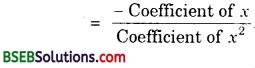and product of zeroes = (- 2)(4) = – 8 = $$\frac{-8}{1}$$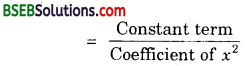2. We have:
4s2 – 4s + 1 = 4s2 – 2s – 2s + 1
= 2s(2s – 1) – 1(2s – 1)
= (2s – 1)(2s – 1)
The value of 4s2 – 4s + 1 is 0, when the value of (2s – 1)(2s – 1) is 0, i.e., when 2s – 1 = 0 or 2s – 1 = 0, i.e.,
when s = $$\frac{1}{2}$$ or s = $$\frac{1}{2}$$.
∴ The zeroes of 4s2 – 4s + 1 are $$\frac{1}{2}$$ and $$\frac{1}{2}$$.
Therefore, sum of the zeroes = $$\frac{1}{2}$$ + $$\frac{1}{2}$$ = 1 = $$\frac{-(-4)}{4}$$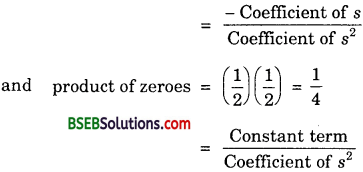3. We have:
6x2 – 3 – 7x = 6x2 – 7x – 3
= 6x2 – 9x + 2x – 3
= 3x(2x – 3) + 1(2x – 3)
= (3x + 1)(2x – 3)
The value of 6x2 – 3 – 7x is 0, when the value of (3x + 1)(2x – 3) is 0, i.e; when 3x + 1 = 0 or 2x – 3 = 0, i.e;
when x = – $$\frac{1}{3}$$ or x = $$\frac{3}{2}$$.
∴ The zeros of 6x2 – 3 – 7x are – $$\frac{1}{3}$$ and $$\frac{3}{2}$$.
Therefore, sum of the zeros = – $$\frac{1}{3}$$ + $$\frac{3}{2}$$ = $$\frac{7}{6}$$ = $$\frac{-(-7)}{6}$$4. We have:
4u2 + 8u is 0, when the value of 4u(u + 2) is 0, i.e; when u = 0 or u + 2 = 0, i.e; when u = 0 or u = – 2.
∴ The zeroes of 4u2 + 8u are o and – 2.
Therefore, sum of the zeroes = 0 + (- 2) = – 2 = $$\frac{-8}{4}$$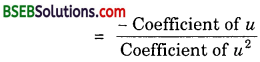and product of zeroes = (0)(- 2) = 0 = $$\frac{0}{4}$$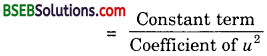5. We have:
t – 15 = (t – $$\sqrt{15}$$)(t + $$\sqrt{15}$$)
The value of t2 – 15 is 0, when the value of (t – $$\sqrt{15}$$)(t + $$\sqrt{15}$$) is 0, i.e; when t – $$\sqrt{15}$$ = 0 or t + $$\sqrt{15}$$ = 0,
i.e; when t = $$\sqrt{15}$$ or t = – $$\sqrt{15}$$.
∴ The zeroes of t2 – 15 are $$\sqrt{15}$$ and – $$\sqrt{15}$$.
Therefore, sum of the zeroes = $$\sqrt{15}$$ + (- $$\sqrt{15}$$) = 0and product of the zeroes = ($$\sqrt{15}$$)(-$$\sqrt{15}$$)
= – 15 = $$\frac{-15}{1}$$6. We have:
3x2 – x – 4 = 3x2 + 3x – 4x – 4
= 3x(x + 1) – 4(x + 1)
= (x + 1)(3x – 4)
The value of 3x2 – x – 4 is 0, when the value of (x + 1)(3x – 4) is 0, i.e; when x + 1 = 0 or 3x – 4 = 0, i,e; when x = – 1 or x = $$\frac{4}{3}$$.
∴ The zeroes of 3x2 – x – 4 are – 1 and $$\frac{4}{3}$$.
Therefore, sum of the zeroes = – 1 + $$\frac{4}{3}$$ = $$\frac{-3+4}{3}$$
= $$\frac{1}{3}$$ = $$\frac{-(-1)}{3}$$and product of the zeroes = (- 1)($$\frac{4}{3}$$) = – $$\frac{4}{3}$$ = $$\frac{-4}{3}$$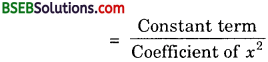Question 2.
Find a quadratie polynomial each with the given numbers as the sum and product of its zeroes respectively.

1. $$\frac{1}{4}$$, – 1
2. $$\sqrt{2}$$, $$\frac{1}{3}$$
3. 0, $$\sqrt{5}$$
4. 1, 1
5. – $$\frac{1}{4}$$, $$\frac{1}{4}$$
6. 4, 1

Solution:
1. Let the polynomial be ax2 + bx + c, and its zeroes be α and β. Then,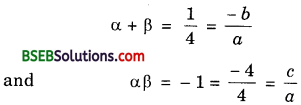If a = 4, then b = – 1 and c = – 4.
∴ One quadratic polynomial which fits the given conditions is 4x2 – x – 4.

2. Let the polynomial be ax2 + bx + c, and its zeroes be α and β. Then,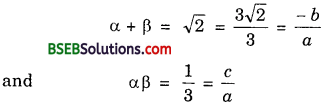If a = 3, then b = – 3$$\sqrt{2}$$ and c = 1.
∴ One quadratic polynomial which fits the given conditions is 3x2 – 3$$\sqrt{2x}$$ + 1.

3. Let the polynomial be ax2 + bx + c, and its zeroes be α and β. Then,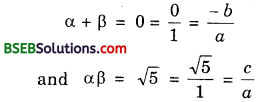If a = 1, then b = 0 and c = $$\sqrt{5}$$.
∴ One quadratic polynomial which fits the given conditions is x2 – 0. x + $$\sqrt{5}$$, i.e; x2 + $$\sqrt{5}$$.

4. Let the polynomial be ax2 + bx + c, and its zeroes be α and β. Then,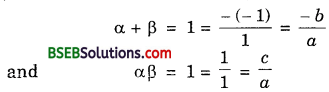If a = 1, then b = – 1 and c = 1.
∴ One quadratic polynomial which fits the given conditions is x2 – x + 1.

5. Let the polynomial be ax2 + bx + c and its zeroes be α and β. Then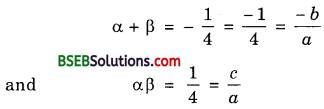If a = 4, then b = 1 and c = 1.
∴ One quadratic polynomial which fits the given conditions is 4x2 + x + 1.

6. Let the polynomial be ax2 + bx + c and its zeroes be α and β. Then,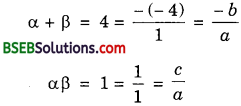If a = 1, then b = – 4, and c = 1.
∴ One quadratic polynomial which fits the given conditions is x2 – 4x + 1.